Maths-
General
Easy

Question

# A can do a piece of work in 40 days and B in 45 days. They work together for 10 days and then B goes away. In how many days will A finish the remaining work?Hint:

## The correct answer is: 21 1/9 days.

### Let’s say that the total work can be represented as W and let’s say the amount of work that A can do in one day is WA and the amount of work that B can do in one day is WB.Step 1 of 3:It is given that A can do the whole work(W) in 40 days.So, work done by A in one day (WA)=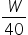……(1)It is given that B can do the whole work(W) in 45 days.So, work done by B in one day (WB)=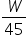……(2)Step 2 of 3:It is given that A and B worked together for 10 days and then B goes away.So, work done by A and B in 10 days = WA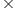10 + WB10=10 +10=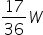Work left for A after B goes away = W - work done by A and B in 10 days= W -=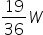Step 3 of 3:From equation (1) time taken by A to completework = 1 daytime taken by B to complete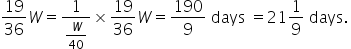Final Answer: Hence, the time taken by B to complete the work after A after 2 days is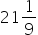days.#### With Turito Foundation.#### Get an Expert Advice From Turito.